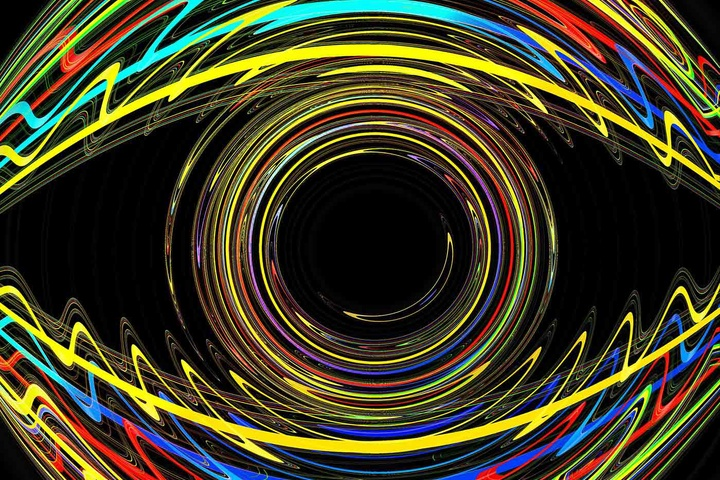model = vgg16.VGG16(weights=’imagenet’, include_top=False)

model.summary()

loss = K.mean(layer_output[:, :, :, neuron_idx])

img_data = np.random.uniform(size=(1, 256, 256, 3)) + 128.

for i in range(16):Notebook版本的代码可以在这个GitHub里找到。脚本化的版本可以在GitHub里找到。Eye (source: Pixabay)